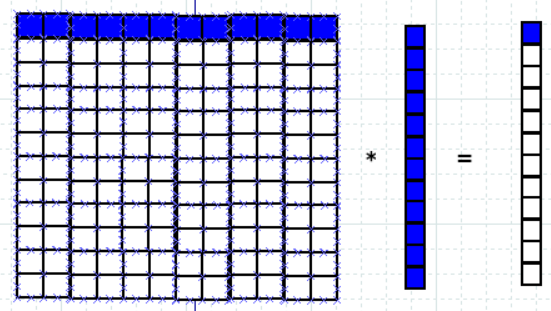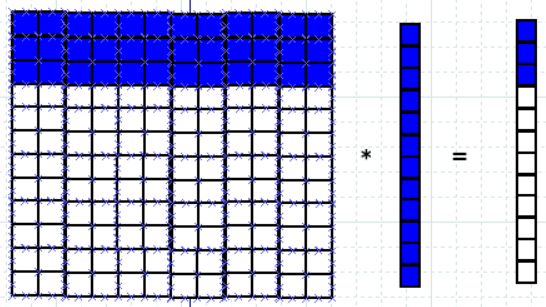## Tuesday, 28 July 2020

### Parallel Computing Terminologies

In this video, we will see Parallel Computing Terminologies: Granularity, Concurrency, Task Dependency Graph, Decomposition, Fine Coarse Grained,  Critical Path | granularity, concurrency, dependency graph, decomposition, task, task dependency graph, fine grained decomposition, coarse grained decomposition, concurrency, maximum degree of concurrency, average degree of concurrency, critical path, critical path length, speedup, task interaction graph, terminologies, parallel computing, high performance computing

Watch this video to know about various terminologies in Parallel Computing or High Performance Computing:

Following are the terminologies used in Parallel Computing:

Reference:
Introduction to Parallel Computing, Second Edition, by Ananth Grama , Anshul Gupta , George Karypis , Vipin Kumar.

1. Decomposition
The process of dividing a computation into smaller parts, some or all of which may potentially be executed in parallel, is called decomposition.

computation into which the main computation is subdivided by means of decomposition.

A task-dependency graph is a directed acyclic graph in which the nodes represent tasks and the directed edges indicate the dependencies amongst them.
The task corresponding to a node can be executed when all tasks connected to this node by incoming edges have completed.
Task-dependency graphs can be disconnected and the edge set of a task-dependency graph can be empty e.g. in Task Dependency Graph of Vector Matrix multiplication, there are no edges as all computations are independent.

e.g. Task Dependency Graph for "Finding minimum element among 23,12,9,30,37,27,8,17":

4. Granularity
The number and size of tasks into which a problem is decomposed determines the granularity
of the decomposition.

Smaller Granularity:Larger GranularityNote: Concurrency usually increase as the granularity of tasks becomes smaller (finer).

5. Fine Grained Decomposition
A decomposition into a large number of small tasks is called fine-grained.6. Coarse Grained Decomposition
A decomposition into a small number of large tasks is called coarse-grained.7. Concurrency
Executing multiple tasks simultaneously, is called concurrency.

8. Maximum Degree of Concurrency
The maximum number of tasks that can be executed simultaneously in a parallel program at any given time is known as its maximum degree of concurrency.
In most cases, the maximum degree of concurrency is less than the total number of tasks due to dependencies among the tasks.
In general, for task dependency graphs that are trees, the maximum degree of concurrency is always equal to the number of leaves in the tree.

e.g.

In above example, Maximum Degree of Concurrency is 4.

9. Average Degree of Concurrency
It is the average number of tasks that can run concurrently over the entire duration of execution of the program.

Note: Both the maximum and the average degrees of concurrency usually increase as the granularity of tasks becomes smaller (finer).

10. Critical Path
A feature of a task-dependency graph that determines the average degree of concurrency for a given granularity is its critical path.
In a task-dependency graph, let us refer to the nodes with no incoming edges by start nodes and the nodes with no outgoing edges by finish nodes. The longest directed path between any pair of start and finish nodes is known as the Critical Path.

11. Critical Path Length
The sum of the weights of nodes along critical path is known as the critical path length, where the weight of a node is the size or the amount of work associated with the corresponding task.

Average Degree of Concurrency = Total Amount of Work / Critical Path Length

e.g. 1.

Total Amount of Work=63
Critical Path Length=27
Average Degree of Concurrency=63/27=2.33

e.g. 2.

Total Amount of Work=64
Critical Path Length=34
Average Degree of Concurrency=64/34=1.88

12. Speedup

Speedup = Serial Execution Time / Parallel Execution Time